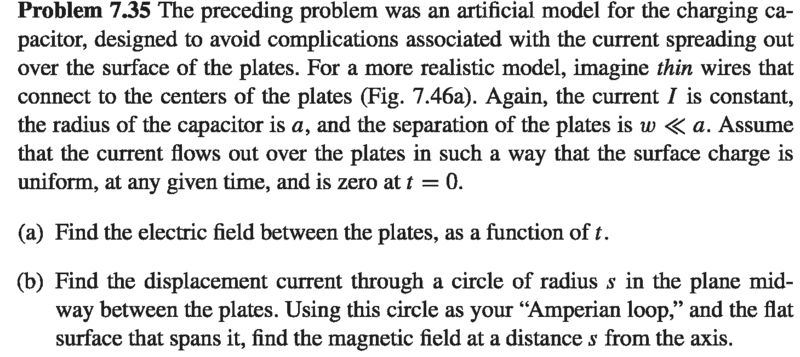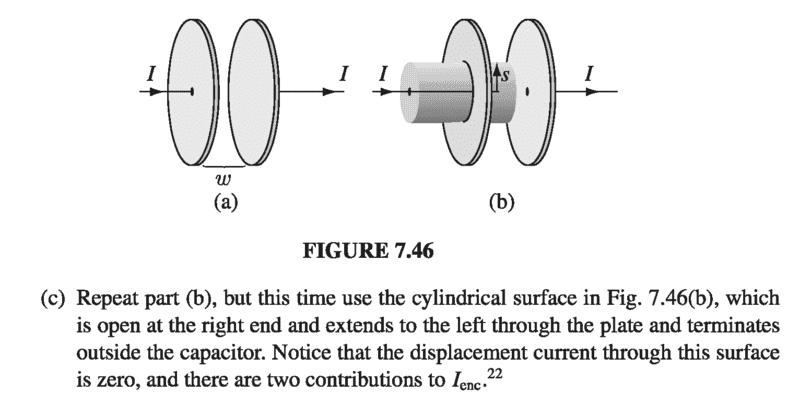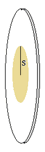# B-field inside a charging || plate (disc shaped) capacitor

## Homework Statement## The Attempt at a Solution

I have difficulty in solving part (C).
The surface bounded by the Amperean loop is like an open drum.
The current enclosed by the loop is ## I_{en}## = I through the bottom of the drum + I(s) through that part of the curved surface which touches the plate .
Now, how to find out I(s)?
How to find out the direction of B considering this surface?
If I consider the planar surface through the Amperean loop, then the displacement current is along z-axis and so the B-field is along the ## \hat \phi ## direction.

Charles Link
Homework Helper
Gold Member
2020 Award

## Homework Statement

View attachment 210261View attachment 210262

## The Attempt at a Solution

I have difficulty in solving part (C).
The surface bounded by the Amperean loop is like an open drum.
The current enclosed by the loop is ## I_{en}## = I through the bottom of the drum + I(s) through that part of the curved surface which touches the plate .
Now, how to find out I(s)?
How to find out the direction of B considering this surface?
If I consider the planar surface through the Amperean loop, then the displacement current is along z-axis and so the B-field is along the ## \hat \phi ## direction.
I think for part "c" they are simply trying to show you the more complete Maxwell's law (in differential form) ## \nabla \times B=\mu_o J+\mu_o \epsilon_o \dot{E} ##. Integrating over ## dA ## and using Stokes theorem gives the more complete form of Ampere's law: ## \oint B \cdot dl=\mu_o I +\mu_o \epsilon_o \int \dot{E} \, \cdot dA ##. Since there is no ## E ## from the capacitor outside the capacitor, I think they are just looking for you to evaluate the left side and the first term of the right side to compute ##B ##. ## \\ ## (I believe the displacement current for part (b) is ## I_D=\epsilon_o \int \dot{E} \cdot \, dA ##). ## \\ ## Editing: One item they usually try to show with this exercise in displacement current is, since we have ## \nabla \times E=-\dot{B} ## (which integrates to, along with Stokes theorem) ## \oint E \cdot \, dl=-\dot{\Phi}_m ##, that there might be a corresponding equation of the form ## \nabla \times B=\mu_o \epsilon_o \dot{E} ## which integrates to ## \oint B \cdot \, dl=\mu_o I_D ## where ## I_D= \epsilon_o \int \dot{E} \cdot \, dA ## . We also can have real currents ## I_S ## in which case we have ## \oint B \cdot \, dl =\mu_o I_S ##, so these two equations are combined into the more complete form of Ampere's law: ## \oint B \cdot \, dl=\mu_o(I_S+I_D) ##. ## \\ ## (In differential form, this more complete equation reads ## \nabla \times B=\mu_o J +\mu_o \epsilon_o \dot{E} ##. This more complete form is used to derive the electromagnetic wave equation).

Last edited:
TSny
Homework Helper
Gold Member
Now, how to find out I(s)?Consider the net charge q in the shaded region of radius s. Can you express q in terms of the total charge Q on the entire plate?

Consider the rate of change of q and how it is related to I and I(s).